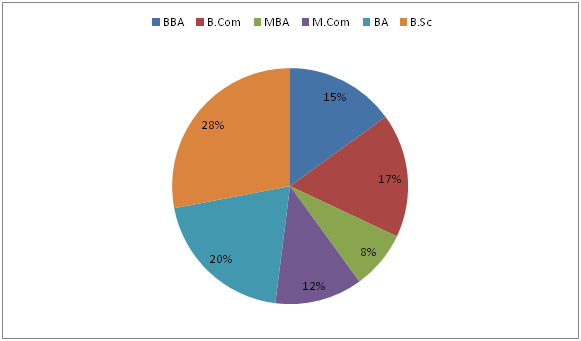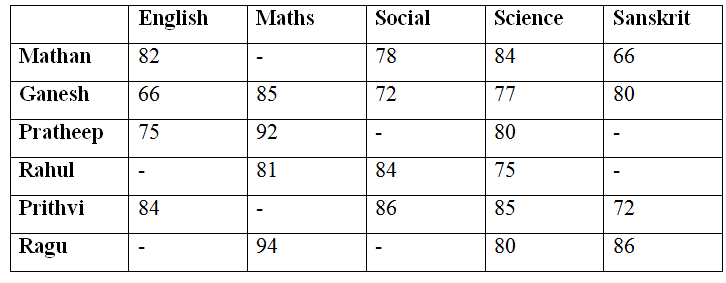# Quantitative Aptitude Questions (Data Interpretation) for RRB Assistant Mains 2018 Day-199

Dear Readers, RRB is conducting Online Examination for the recruitment of Assistant. To enrich your preparation here we have providing new series of Data Interpretation – Quantitative Aptitude Questions. Candidates those who are appearing in RRB Assistant Mains Exams can practice these Quantitative Aptitude average questions daily and make your preparation effective.

[WpProQuiz 3718]

Click “Start Quiz” to attend these Questions and view Solutions

Directions (Q. 1 – 5) Study the following information carefully and answer the given questions:

The following pie chart shows the percentage distribution of total number of students in different departments in a certain college in the year 2018.

Total number of students = 35001) If the ratio between the total number of male to that of female students in BBA is 3 : 2, then find the central angle corresponding to the female students in BBA?

a) 18.80

b) 35.40

c) 21.60

d) 27.20

e) None of these

2) If the total number of male students in MBA is 60 % of total number of students in MBA and the ratio between the total number of male students in MBA to that of total number of female students in B. Sc is 4 : 5, then find the total number of male students in B. Sc?

a) 770

b) 520

c) 480

d) 660

e) None of these

3) If 25 % and 40 % of M. Com and BA students participated in various sports events respectively, then find the total number of students didn’t participate any sports of department of M. Com and BA together?

a) 678

b) 712

c) 656

d) 735

e) None of these

4) Find the difference between the total number of BBA students to that of BA students?

a) 150

b) 175

c) 190

d) 135

e) None of these

5) If the total number of male to that of female students in B. Com is 3 : 2, then the total number of female students in B.Com is what percentage of total number of students in MBA?

a) 75 %

b) 80 %

c) 85 %

d) 90 %

e) None of these

Directions (Q. 6 – 10) Study the following information carefully and answer the given questions:

The following table shows the total marks obtained by 6 different students in 5 different subjects. A maximum mark in each subject is 100.6) Find the marks scored by Prithvi in Maths, if the average marks scored by all the given students in Maths is 87 and the ratio between the marks scored by Mathan to that of Prithvi in Maths is 9 : 8?

a) 80

b) 110

c) 100

d) 90

e) None of these

7) Find the difference between the total marks scored by Science to that of Sanskrit of all the given students together, if the average mark scored by all the given students in Sanskrit is 76?

a) 50

b) 40

c) 65

d) 25

e) None of these

8) Find the percentage of marks scored by Ganesh in all the given subjects together?

a) 82 %

b) 76 %

c) 70 %

d) 85 %

e) None of these

9) Find the ratio between the total marks scored by Pratheep in Sanskrit to that of Prithvi in Maths, if the ratio between the marks scored by Mathan and Pratheep in Social is 6 : 5 and the total marks scored by Pratheep in Social, Science and Sanskrit is 219 and the average marks scored by Prithvi in Maths and Social is 83?

a) 37 : 40

b) 18 : 23

c) 25 : 32

d) 8 : 17

e) None of these

10) Total marks scored by Ragu in Science and Sanskrit together is what percentage of total marks scored by Mathan in English and Social together?

a) 92.25 %

b) 88.5 %

c) 103.75 %

d) 112.25 %

e) None of these

Direction (1-5) :

Explanation:

The ratio between the total number of male to that of female students in BBA = 3 : 2

The central angle corresponding to the female students in BBA

= > 360*(15/100)*(2/5)

= > 21.60

Explanation:

The total number of male students in MBA

= > 60 % of total number of students in MBA

= > (60/100)*3500*(8/100) = 168

The ratio between the total number of male students in MBA to that of total number of female students in B. Sc = 4 : 5 (4x, 5x)

4x = 168

X = 42

The total number of female students in B. Sc = 5x = 210

The total number of students in B. Sc = 3500*(28/100) = 980

The total number of male students in B. Sc = 980 – 210 = 770

Explanation:

The total number of students didn’t participate any sports of department of M. Com and BA together

= > 3500*(12/100)*(75/100) + 3500*(20/100)*(60/100)

= > 315 + 420 = 735

Explanation:

The total number of BBA students

= > 3500*(15/100) = 525

The total number of BA students

= > 3500*(20/100) = 700

Required difference = 700 – 525 = 175

Explanation:

The total number of male to that of female students in B. Com = 3 : 2

The total number of female students in B.Com

= > 3500*(17/100)*(2/5) = 238

Total number of students in MBA = 3500*(8/100) = 280

Required % = (238/280)*100 = 85 %

Direction (6-10) :

Explanation:

The average marks scored by all the given students in Maths = 87

The total marks scored by all the given students in Maths = 87*6 = 522

The ratio between the marks scored by Mathan to that of Prithvi in Maths = 9 : 8 (9x, 8x)

The total marks scored by Mathan and Prithvi in Maths

= > 522 – (85 + 92 + 81 + 94)

= > 522 – 352 = 170

According to the question,

9x + 8x = 170

17x = 170

X = 10

The marks scored by Prithvi in Maths = 8x = 80 marks

Explanation:

The total marks scored by Science of all the given students together

= > 84 + 77 + 80 + 75 + 85 + 80 = 481

The average marks scored by all the given students in Sanskrit = 76

The total marks scored by Sanskrit of all the given students together

= > 76*6 = 456

Required difference = 481 – 456 = 25

Explanation:

The total marks scored by Ganesh in all the given subjects together

= > 66 + 85 + 72 + 77 + 80 = 380

The percentage of marks scored by Ganesh in all the given subjects together

= > (380/500)*100 = 76 %

Explanation:

The ratio between the marks scored by Mathan and Pratheep in Social = 6 : 5

6x = 78

X = 13

The marks scored by Pratheep in Social = 65

Total marks scored by Pratheep in Social, Science and Sanskrit = 219

The marks scored by Pratheep in Sanskrit = 219 – (65 + 80) = 74

The average marks scored by Prithvi in Maths and Social = 83

The marks scored by Prithvi in Maths = (83*2) – 86 = 80

Required ratio = 74 : 80 = 37 : 40

Explanation:

Total marks scored by Ragu in Science and Sanskrit together

= > 80 + 86 = 166

Total marks scored by Mathan in English and Social together

= > 82 + 78 = 160

Required % = (166/160)*100 = 103.75 %

Daily Practice Test Schedule | Good Luck

 Topic Daily Publishing Time Daily News Papers & Editorials 8.00 AM Current Affairs Quiz 9.00 AM Quantitative Aptitude “20-20” 11.00 AM Vocabulary (Based on The Hindu) 12.00 PM General Awareness “20-20” 1.00 PM English Language “20-20” 2.00 PM Reasoning Puzzles & Seating 4.00 PM Daily Current Affairs Updates 5.00 PM Data Interpretation / Application Sums (Topic Wise) 6.00 PM Reasoning Ability “20-20” 7.00 PM English Language (New Pattern Questions) 8.00 PM